# Ostwald Ripening

The Easy Part and the ProblemOstwald ripening, sometimes called "particle coarsening" is very easy to understand within the context of the second law. For binary A - B materials like the iron - carbon system, the second law may demand mixed phases (like ferrite - cementite) for certain conditions. If we look at cases where the concentration of the constituent B (e.g. carbon) is small (e.g. 1% carbon), we thus must have B containing precipitates (e.g. cementite, Fe3C) in an A matrix (e.g. ferrite or Fe + a tiny amount of dissolved carbon).The second law demands mixed phases if that is necessary for having the state of lowest free enthalpy or simply energy. For exactly the same reasons it is then necessary to have the largest possible precipitates because this minimizes the interphase energy between precipitate and matrix.
So much for the ideal world of the second lawIn a real world precipitation is difficult to start, and precipitates are typically small. The system is thus not at nirvana proper. It does not have the lowest possible energy and thus will try to get there by doing whatever is necessary. Obviously it is necessary to move from having many small precipitates to having only a few big ones. This can only happen in a continuous process where small ones shrink and large ones grow, and this will take some time.
This process is called "Ostwald ripening".That Ostwald ripening should occur is thus easy to predict. It is also easy to predict that Ostwald ripening must be a gradual process, increasing the average size of precipitates and decreasing their total number continuously as a function of time. With a bit of thought is also clear that diffusion, the movements of atoms inside the material, will be essential to the process. It thus might be so sluggish at low temperatures that it will not be observed within reasonable time spans.So far, so easy. Now let's turn to the difficult part. As it turn out, it is rather difficult to calculate exactly how Ostwald ripening proceeds. Big names have tried and failed.
Just for fun I show you how to start: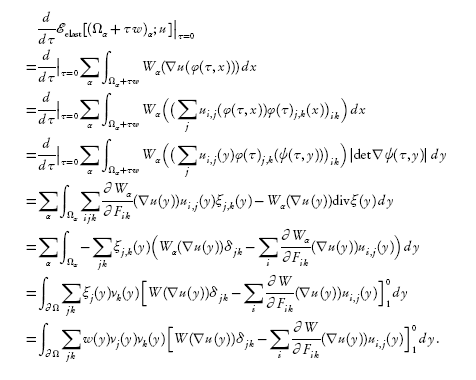How you start to calculate Ostwald ripening Source: Stefan Reiß; "Theorie und Computersimulation der Ostwald-Reifung bei der Ionenstrahlsynthese" PhD thesis, Uni Dresden, GermanyI'll beg your pardon for not going into details. In what follows I will just give some of the essentials and the "final" solution for cases of interest to us.

Getting StartedYou may or may not remember that once upon a time I discussed the nucleation of precipitates and argued (convincingly) that a precipitate must have a certain critical size rcrit(start) before it can start to grow in an environment where there is some supersaturation of some atomically dissolved constituent (like carbon in iron) and therefore a driving force for precipitation.
The basic equation obtained was

rcrit(start)   =  2g/DgThe two important parameters are g = specific interface energy (energy per area; unit: J/m2) and Dg, the specific energy gained per particle (volume) if it is put into a precipitate. It is thus directly the driving force for the precipitation reaction.
The precipitate must have a radius equal to or larger than the critical radius given above if it is to start growing. If it is smaller it will tend to shrink. Since Dg depends on temperature, a particle that is happily growing at one temperature might find itself to shrink at another temperature, and vice verse,.
Now would be a good time to take a quick look at the nucleation modules.

 Science Module NucleationI am now going to argue that there is another (much larger) critical radius rcrit(stop) that causes the precipitate to stop growing and to start shrinking. That's the starting point for looking at Ostwald ripening.
That should come as surprise because it is not as obvious as the critical radius rcrit(start) needed for starting growth. There is indeed no reason for a (large) critical growth-stop radius rcrit(stop) if we only consider one precipitate AnBm in some matrix A with supersaturated B point defects as we did for rcrit(start). We need to look at several precipitates, at least two, that interact with the matrix and via the matrix with each other.
The starting point for doing this is the same as always. We know that a precipitate only grows if that helps to lower the "free enthalpy" of the system. Running through the numbers, one precipitate exceeding the critical radius rcrit(start) then grows as long as B atoms are supersaturated. But that is just the basic condition and applies without reservation only to a system with only one precipitate. Now consider a system with two precipitates; one a bit smaller than the other. For lowering the energy it would be enough if just one of the to precipitates grows, gobbling up all the surplus atoms. It would be even better if only one precipitate is left in the end, then the other one would actually need to shrink. We thus cannot automatically expect that both precipitates will grow. Nothing helps, we need to consider the coupled growth and shrinkage of at least two individual precipitates inside some system A with supersaturated B impurity atoms.
The crucial step is to realize that a precipitate, that is embedded in a "sea" of randomly diffusing impurity atoms, will only grow if there is a net diffusion current of impurity atoms that impinges on the surface of the precipitates (which is, of course, the interface with the matrix). When I write "current" I mean as always the particle current density measured in atoms per second and square-centimeter (or better square-nanometer) that impinges per second on an area increment of the precipitate. The postulated existence of a (positive) net current necessitates two conditions:1. Any diffusion current flowing towards the precipitate needs a concentration gradient with a decreasing concentration from the bulk towards the precipitate surface. That is the statement of Fick's first law. Read up on it in the link if you forgot about that.
That the concentration of impurities decreases in the neighborhood of a growing precipitate is easy to see: If the surface immediately incorporates every atom arriving on it into the precipitate, the concentration close to the surface would be close to zero. If your son immediately spends all the money that is making it from your purse or account (with a large concentration of money inside) to him, the concentration in his purse / account will always be close to zero.
2. A net diffusion current needs a larger current flowing into the precipitate than the one that flows out of it.
Huh? Why should there be B-atoms flowing away from the precipitate? Because precipitates are stupid. They don't know about the second law. They just grab suitable atoms coming by and bind them with some "force" or better energy, onto their surface. The binding energy is never so large, however, that none of these atoms will ever be able to escape again. Some will always manage to rip themselves off, running away from the precipitate into the bulk.All we need to do now is to recognize that the ripping-off process is easier for small precipitates than for large ones. Why is that? Let's look at a simplified model that contains the essentials to get an idea. Imagine a sphere made from square (or round) building blocks of finite size like atoms or molecules. It then looks like that: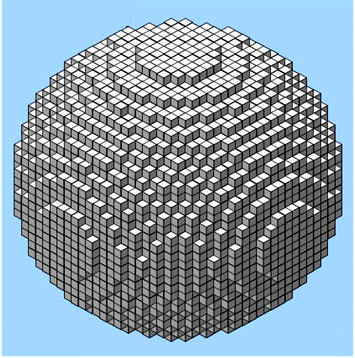Sphere made from cubes Source: Internet at largeThe cubes (= atoms) at the ridges are not as tightly bound to the precipitate than the others, they simply have fewer neighbors to attach to. These are thus the atoms that could most easily break loose again. Since a small sphere has obviously far more ridges (per area increment) than a large one, it will emit more B-atoms (per area increment) than a large one.
Easy to see, not so easy to calculate.In a simple one-precipitate theory, the precipitate would stop growing as soon as the B-concentration at its surface would be equal to the bulk equilibrium concentration cB, equ(bulk). The long and short of all the calculations that we now would need to do for two or more precipitates is that a precipitate with radius r stops growing for concentrations cB(r), i.e. the concentration of B atoms right on its surface, that are larger than the equilibrium bulk concentration cB, equ(bulk). How much larger depends on the size of the precipitate as given by its radius r. An individual precipitate is at equilibrium (= not changing anymore) as soon as the bulk concentration is the same as this r-dependent surface concentration, so we may call the "growth-stop" concentration just as well "equilibrium concentration" cB, equ(r). For a very large precipitates the difference between the "stop" concentration and the equilibrium bulk concentration is very small, but for small precipitates it can be substantial. The reason for this is the higher emission rate for small precipitates.This is now easy to illustrate schematically. What we will have in terms of concentrations of B in A at some temperature T in the vicinity of two precipitates with radii r1 and r2 > r1 is shown below: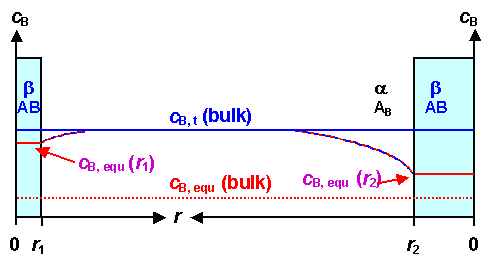Concentration of precipitating species B in the bulk of A and around precipitatesWe have a small precipitate to the left, and a larger one to the right. On the concentration axis we have (in order of appearance):
• Blue line: The actual concentration of B in the in the matrix A; cB, t(bulk) at some time t. In the figure B is supersaturated. cB, t(bulk) is a number that changes with time and bottoms out at the equilibrium concentration.
• Solid red line inside precipitates: The growth-stop or equilibrium concentration cB, equ(r) of B in A right next to the interface between the precipitate and the A matrix phase. It depends on the size r oft the precipitate and is a fixed value for a fixed size. It does change in time if the size of the precipitate changes with time. The larger the precipitate, the closer it gets to:
• Dashed red line: The equilibrium (bulk) concentration of B in A; cB, equ(bulk) at the given temperature as given by the phase diagram. Example: The equilibrium concentration of carbon in ferrite at room temperature is cC in Fe(equ; RT) = 0.008 wt%. That is the concentration that the system tries to achieve by growing precipitates that allow to lower cB, equ(t) eventually to cB, equ(bulk).
• Dashed blue-red line: The concentration change of B from the precipitate to the bulk, defining the concentration gradient necessary for a diffusion current.In the picture above both precipitates will grow because their individual equilibrium concentrations at the interface are lower than the actual bulk concentrations. The figure shows that graphically: There is a downhill concentration profile in both cases, causing B atoms to diffuse towards the precipitate.Now let's look what will happen to the system above at some later time. Both precipitates will have grown somewhat, and the concentration of B in A (C in ferrite) will therefore be closer to the equilibrium bulk concentration: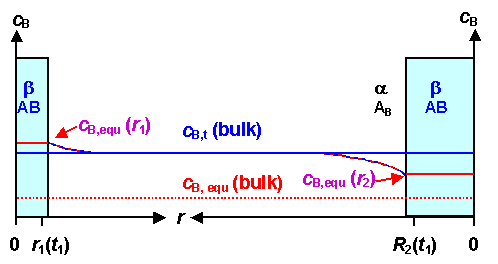Concentration of precipitating species in the bulk and around precipitates as above but a little laterThe bigger precipitate will still grow since it still has a "downhill" concentration profile. But the smaller precipitate will now shrink; the concentration profile around it is now "uphill". Remember, that the net diffusion current is always directly tied to the gradient of the concentration!
At the moment when the bulk concentration was identical to its "personal" equilibrium concentration cB, equ(r), it had no more reason to grow. If the bulk concentration goes below that value, it has energetic reasons to shrink. Kinetically, this simply happens because the net diffusion current will now point away from the precipitate; more B-atoms get emitted than absorbed.Here we have the essence of Ostwald ripening. "Small" precipitates might shrink under certain circumstances, while larger ones still grow.
Note that so far I have not invoked the interface energy g. The whole discussion above only concerned Dg > 0, the energy gain resulting by putting B atoms into an AB precipitate.
If we look again at the derivation of the critical "go" radius we (should) realize that the result as given above also holds for the critical "stop" radius. All we have to do now is to find an expression for the dependence of Dg on the various concentrations and the temperature T.In the nucleation module I gave you an expression for Dg. But that was only approximate and for special cases. The assumption was that Dg is proportional to the difference between melting point temperature and actual temperature. That is far too simple for what we have in mind here.Getting a suitable expression is not all that difficult but well beyond the scope of what I'm doing here. So I will only give the result:

Dg  =  kT · cB, prec · ln cB, t(bulk)
cB, equ(r)The quantity cB, prec is the concentration of the B-atoms in the precipitate. For example, the concentration of carbon atoms in a Fe3C precipitate is cC, Fe3C = 0.25 at%.
The ratio cB, t(bulk)/cB, equ(bulk) is simply the supersaturation of the B atoms as seen from a precipitate with radius r.Dg is the driving force for the reaction. For big precipitates and then cB, equ(r ® ¥) = cB, equ(bulk). Dg it becomes zero, as one would expect, as soon as cB, t(bulk) = cB, equ(bulk).
However, for small precipitates the driving force becomes zero as soon as cB, t(bulk) = cB, equ(r) > cB, equ(bulk)..
In other words: A small precipitate will stop growing before the bulk equilibrium concentration is reached. If the concentration goes below its equilibrium value, it is induced to shrink.It is now easy to calculate the critical radius for growth or shrinkage - same thing. And we don't have to distinguish between the critical radius for nucleation and growth-stop anymore. It's all the same now and a function of the various parameters involved, including time.
All we have to do is to insert the Dg equation into the critical radius equation, yielding:

rcrit =

2g
kT · cB, prec · ln  cB, t(bulk)
cB, equ(r)This is a rather tricky equation. It implies, for example, that the critical radius increases with decreasing temperature, while our old equation from only nucleation implies that the critical radius decreases with decreasing temperature. The reason is that for nucleation only we considered just one to-be-formed precipitate in a sea of supersaturated B-atoms for any temperature. As the temperature goes down, the supersaturation goes up dramatically as long as no precipitate has formed.
But now we have a defined supersaturation that shouldn't be too large for the assumptions made, and consider one existing precipitate in a "sea" of other precipitates and a mild supersaturation of B-atoms.
In principle, the equation even allows rcrit to decrease with decreasing temperature - as long as the ln of the supersaturation goes up faster that the temperature goes down. That will only happen close to a transition temperature, if at all.Let's just look what all of the above implies in a schematic way: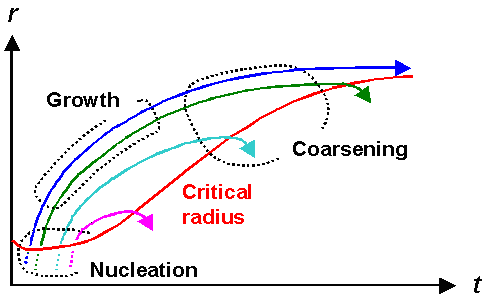Nucleation, growth and shrinkage of precipitates as a function of time Source: "Werkstoffkunde Stahl"Shown is a typical development of precipitates during cooling down. The red line shows the typical behavior of the critical radius for some cooling rate as a function of time. For short times in the beginning it might actually decrease somewhat but after the first precipitates managed to nucleate it will increase since the supersaturation comes down.
The other solid lines show the schematic fate of various precipitates. After nucleation they all grow, i.e. their radius increases with time. All but one, however, will "hit " the red line after some growth period, meaning that they have reached the critical radius for shrinkage. They thus will start to shrink, releasing B atoms, and this effectively feeds the "blue" precipitate, the only one in this figure that manages to grow faster than the critical radius.It should be clear by now why the math for Ostwald ripening is difficult. Everything depends on everything else. What the precipitates do determines the shape of the red line, and that determines what the precipitates do...
So let's stop here with looking at the basics and only consider how Ostwald ripening will express itself in practical cases.This is relatively simple. After long and assiduous calculations, involving a lot of assumptions, the final result is that the average size of precipitates (or their radius r) in halfway normal metals (like iron) increases with the third root of time, i.e. with t1/3 if the system is held at some constant temperature T.
In other words:

r  =  A · t1/3
A  =  const  µ  D · g
T

So large interface energies g quicken the ripening process, and as always, it scales with the diffusion coefficient D. If atoms can hardly move (very small D) nothing much happens. Note that D decreases exponentially with decreasing T so the constant A decreases with decreasing T even so T appears in the denominator.
The following data illustrate that the "t1/3 law" is sound: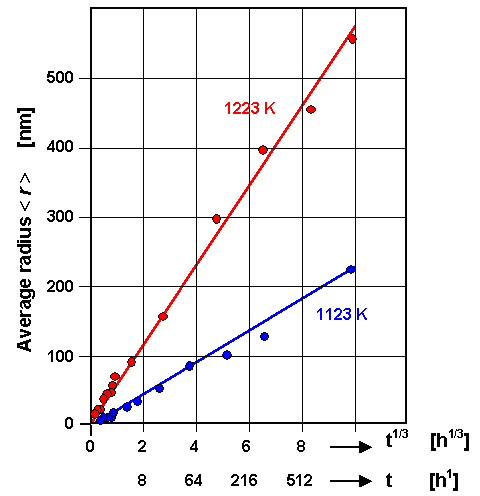Ostwald ripening of precipitates in a in a Ni base alloy Source: B, Ilschner; "Werkstoffwissenschaften", Springer Verlag 1990The t1/3 law is nicely demonstrated. Moreover, it becomes clear once more that temperature is essential.
Why don't I show steel data? Well I haven't found nice ones. If you consider what it takes to obtain data like this (besides grad students), you understand that there are not too many measurement around that are as nice as the one above.As a last thought I want to mention that particle coarsening can be sped up a lot if you oscillate the temperature around a phase transition temperature with a high temperature phase that does not need precipitates. Take the g Û a in iron as example. What you do is:
1. Keep above A1 for a while. All the carbon is dissolved.
2. Go to somewhat below the transition temperature A1. Precipitation should occur but nucleation is difficult. Precipitation eventually starts statistically at different times in different places. You get precipitates with different sizes, all growing. Keep then growing or some time.
3. Go somewhat above A1 for some time. All precipitates shrink; the smaller ones will completely disappear.
4. Go below A1 again before all precipitates have dissolved. Precipitation should occur again but now nucleation is easy since there are already some precipitates - the survivors from the bigger ones formed in the step before - that simply grow again.
5. Go somewhat above A1 for some time....
In the end, only the really large precipitates from the first step will have survived; no small ones are around anymore.Easy in principle but a bit tricky in reality. You must get the "somewhat" above or below A1 and the some time just right if the recipe is supposed to work. It's not all that easy to figure that out by experiments. Once more, the first law of applied science rears its beautiful head!If you call that process still "Ostwald ripening" is a matter of taste. We will encounter it as soon as we look at Wootz steel.Books and Other Major SourcesDiffusion in IronNucleation Science7.1.1 Finding Your Way in the Iron Carbon Phase DiagramTTT Diagrams: 1. The Basic Idea11.5 Wootz Swords; 11.5.1 The Winner is....The Al - Cu System11.5.2 Structure by Dendrites?11.5.3 Forging a Wootz SwordBlooms and Bloomeries4.4.1 Perfect Crystals and the Second LawPhenomenological Modelling of Diffusion8.2.2 Tempering and Ostwald Ripening6.1.1 It Takes Two to TangoIntrinsic Gettering in SiliconThe Second LawThe Goldilocks PrincipleHomogeneous NucleationTTT Diagrams 4. Experimental Construction of TTT and Phase Diagrams

© H. Föll (Iron, Steel and Swords script)